Open in App
Not now

# Arithmetic Operations on Images using OpenCV | Set-2 (Bitwise Operations on Binary Images)

• Difficulty Level : Easy
• Last Updated : 04 Jan, 2023

Prerequisite: Arithmetic Operations on Images | Set-1
Bitwise operations are used in image manipulation and used for extracting essential parts in the image. In this article, Bitwise operations used are :

1. AND
2. OR
3. XOR
4. NOT

Also, Bitwise operations helps in image masking. Image creation can be enabled with the help of these operations. These operations can be helpful in enhancing the properties of the input images.
NOTE: The Bitwise operations should be applied on input images of same dimensions
Input Image 1:Input Image 2:### Bitwise AND operation on Image:

Bit-wise conjunction of input array elements.

Parameters:
source1: First Input Image array(Single-channel, 8-bit or floating-point)
source2: Second Input Image array(Single-channel, 8-bit or floating-point)
dest: Output array (Similar to the dimensions and type of Input image array)

## Python3

 `# Python program to illustrate  ` `# arithmetic operation of ` `# bitwise AND of two images ` `    `  `# organizing imports  ` `import` `cv2  ` `import` `numpy as np  ` `    `  `# path to input images are specified and    ` `# images are loaded with imread command   ` `img1 ``=` `cv2.imread(``'input1.png'``)   ` `img2 ``=` `cv2.imread(``'input2.png'``)  ` ` `  `# cv2.bitwise_and is applied over the ` `# image inputs with applied parameters  ` `dest_and ``=` `cv2.bitwise_and(img2, img1, mask ``=` `None``) ` ` `  `# the window showing output image ` `# with the Bitwise AND operation ` `# on the input images ` `cv2.imshow(``'Bitwise And'``, dest_and) ` `  `  `# De-allocate any associated memory usage   ` `if` `cv2.waitKey(``0``) & ``0xff` `=``=` `27``:  ` `    ``cv2.destroyAllWindows()  `

Output: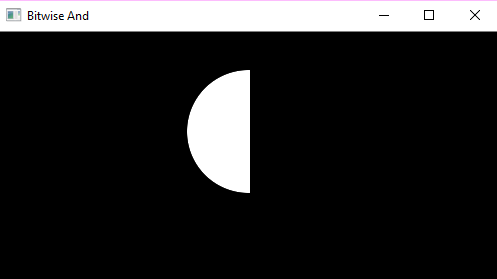### Bitwise OR operation on Image:

Bit-wise disjunction of input array elements.

Parameters:
source1: First Input Image array(Single-channel, 8-bit or floating-point)
source2: Second Input Image array(Single-channel, 8-bit or floating-point)
dest: Output array (Similar to the dimensions and type of Input image array)

## Python3

 `# Python program to illustrate  ` `# arithmetic operation of ` `# bitwise OR of two images ` `    `  `# organizing imports  ` `import` `cv2  ` `import` `numpy as np  ` `    `  `# path to input images are specified and    ` `# images are loaded with imread command   ` `img1 ``=` `cv2.imread(``'input1.png'``)   ` `img2 ``=` `cv2.imread(``'input2.png'``)  ` ` `  `# cv2.bitwise_or is applied over the ` `# image inputs with applied parameters  ` `dest_or ``=` `cv2.bitwise_or(img2, img1, mask ``=` `None``) ` ` `  `# the window showing output image ` `# with the Bitwise OR operation ` `# on the input images ` `cv2.imshow(``'Bitwise OR'``, dest_or) ` `  `  `# De-allocate any associated memory usage   ` `if` `cv2.waitKey(``0``) & ``0xff` `=``=` `27``:  ` `    ``cv2.destroyAllWindows()  `

Output: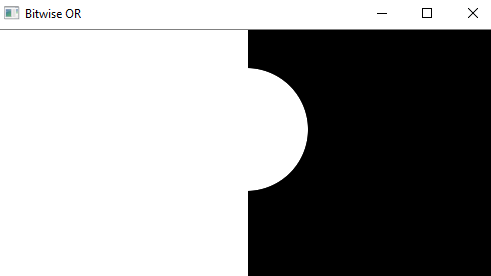### Bitwise XOR operation on Image:

Bit-wise exclusive-OR operation on input array elements.

Parameters:
source1: First Input Image array(Single-channel, 8-bit or floating-point)
source2: Second Input Image array(Single-channel, 8-bit or floating-point)
dest: Output array (Similar to the dimensions and type of Input image array)

## Python3

 `# Python program to illustrate  ` `# arithmetic operation of ` `# bitwise XOR of two images ` `    `  `# organizing imports  ` `import` `cv2  ` `import` `numpy as np  ` `    `  `# path to input images are specified and    ` `# images are loaded with imread command   ` `img1 ``=` `cv2.imread(``'input1.png'``)   ` `img2 ``=` `cv2.imread(``'input2.png'``)  ` ` `  `# cv2.bitwise_xor is applied over the ` `# image inputs with applied parameters  ` `dest_xor ``=` `cv2.bitwise_xor(img1, img2, mask ``=` `None``) ` ` `  `# the window showing output image ` `# with the Bitwise XOR operation ` `# on the input images ` `cv2.imshow(``'Bitwise XOR'``, dest_xor) ` `  `  `# De-allocate any associated memory usage   ` `if` `cv2.waitKey(``0``) & ``0xff` `=``=` `27``:  ` `    ``cv2.destroyAllWindows()  `

Output: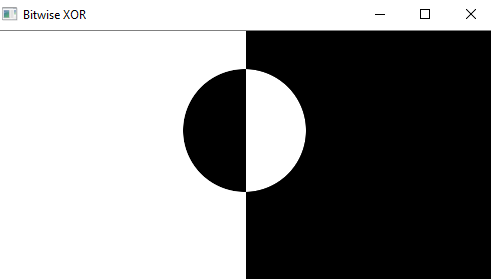### Bitwise NOT operation on Image:

Inversion of input array elements.

Parameters:
source: Input Image array(Single-channel, 8-bit or floating-point)
dest: Output array (Similar to the dimensions and type of Input image array)

## Python3

 `# Python program to illustrate  ` `# arithmetic operation of ` `# bitwise NOT on input image ` `    `  `# organizing imports  ` `import` `cv2  ` `import` `numpy as np  ` `    `  `# path to input images are specified and    ` `# images are loaded with imread command   ` `img1 ``=` `cv2.imread(``'input1.png'``)   ` `img2 ``=` `cv2.imread(``'input2.png'``)  ` ` `  `# cv2.bitwise_not is applied over the ` `# image input with applied parameters  ` `dest_not1 ``=` `cv2.bitwise_not(img1, mask ``=` `None``) ` `dest_not2 ``=` `cv2.bitwise_not(img2, mask ``=` `None``) ` ` `  `# the windows showing output image ` `# with the Bitwise NOT operation ` `# on the 1st and 2nd input image ` `cv2.imshow(``'Bitwise NOT on image 1'``, dest_not1) ` `cv2.imshow(``'Bitwise NOT on image 2'``, dest_not2) ` `  `  `# De-allocate any associated memory usage   ` `if` `cv2.waitKey(``0``) & ``0xff` `=``=` `27``:  ` `    ``cv2.destroyAllWindows()  `

Output:
Bitwise NOT on Image 1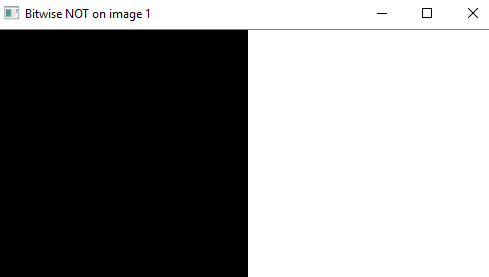Bitwise NOT on Image 2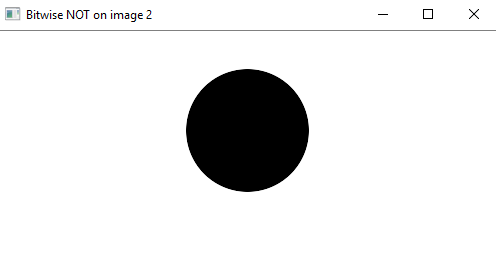My Personal Notes arrow_drop_up
Related Articles